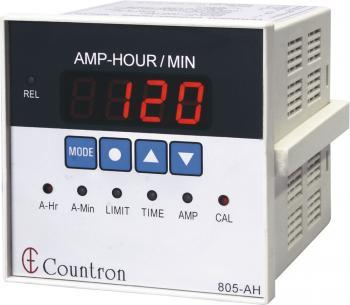Ampere-HourAn ampere-hour is an electric charge unit. It is represented by Ah. The ampere-hour is not part of the International System, since the hour is not either.

The ampere-hour indicates the amount of electrical charge that passes through the terminals of a battery (or an electrical conductor) providing an electric current of 1 ampere (A) for one hour (h).

The ampere-hour is used to measure the capacity of a battery, ie the amount of electricity it can store during charging and return during discharge.

A battery that has, for example, a capacity of 100 Ah, theoretically can give a current of 10 amps for 10 hours, or 1 amp for 100 hours.

On a practical level, there are certain losses of performance conditional on the speed of the battery discharge, the faster a battery is discharged, the more energy is lost through internal resistance. Therefore, the load capacity is usually referred to a standard discharge time (10 or 20 hours), and for a certain final voltage.

The equivalence of an ampere-hour is 3600 Coulombs.

In the electrochemical calculations, the Faraday constant is used. The Faraday constant is the charge in one mole of electrons, approximately equal to 26.8 amps per hour.

Because the ampere-hour is a unit related to electrical energy and batteries, in the field of solar energy its use is limited in the field of photovoltaic solar energy and in large thermal power plants.

Use of the ampere-hour

The Ampere-hour is used in batteries, in solar batteries or in electric batteries in general to indicate the capacity. In automobile batteries, it is maintained that the 12 V battery must be able to supply an electric discharge intensity of 1 / 20th of the rated capacity indicated in A · h for 20 hours at a temperature of 25 ° C at a voltage higher than the cut that is 10.5V.

The ampere-hour is also frequently used in measurements of electrochemical systems, such as electroplating.

The milliampere of a second (mAs) is a unit of measurement used in X-ray images, imaging and radiotherapy. This amount is proportional to the total energy of X-rays produced by a given X-ray tube operated at a particular voltage. The same total dose can be administered in different periods of time depending on the current of the X-ray tube.

valoración: 3.1 - votos 7

Last review: February 21, 2018